Question

If the volume of a cube is 15.625 cubic yards, what is the side length?
A. 5.2 yards
B. 3.95 yards
C. 2.5 yards
D. 5.2 cubic yards
E. 3.95 cubic yards
F. 2.5 yards cubed

1.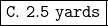Step-by-step explanation:

The volume of a cube can be found using the following formula: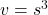By cubing the side length, the volume is found. We know the volume is 15.625 cubic yards, so we can substitute 15.625 yd³ in for v.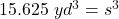We want to find the side length, s. Therefore, we must isolate s on one side of the equation.

s is being cubed. The inverse of a cube is a cube root. Take the cube root of both sides of the equation.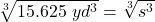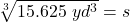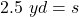Choose the correct answer choice. C. 2.5 yards is correct, but be careful with choice F. Notice that the units for F are cubic yards.

The side length is 2.5 yards and choice C is correct.